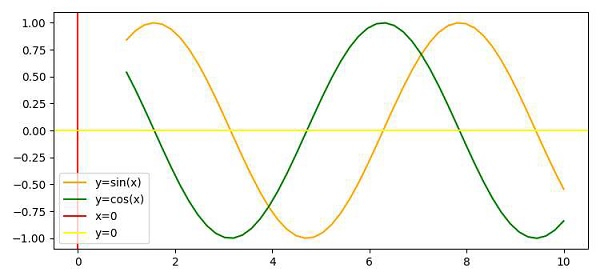# Show the origin axis (x,y) in Matplotlib plot

To show the origin, we can take the following Steps −

• Create the points x, y1 and y2 using numpy.

• Plot the sine and cosine curves using plot() methods.

• Plot the vertical line, i.e., x=0.

• Plot the horizontal line, i.e., y=0.

• Intersection point of (Step 3 and 4), could be the origin.

• To display the label of lines, use legend() method.

• To display the figure, use show() method.

## Example

import numpy as np
from matplotlib import pyplot as plt
plt.rcParams["figure.figsize"] = [7.50, 3.50]
plt.rcParams["figure.autolayout"] = True
x = np.linspace(1, 10, 50)
y1 = np.sin(x)
y2 = np.cos(x)
plt.plot(x, y1, c="orange", label="y=sin(x)")
plt.plot(x, y2, c="green", label="y=cos(x)")
plt.axvline(x=0, c="red", label="x=0")
plt.axhline(y=0, c="yellow", label="y=0")
plt.legend()
plt.show()

## Output